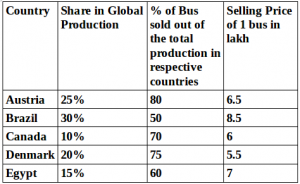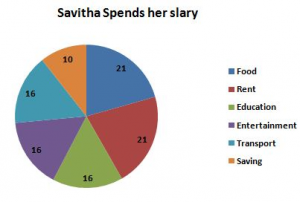## Data Interpretation For SBI PO : Set – 48

D.1-5) Study the following pie-charts and information to answer the given questionsTotal global production is 40000 cars in the given quarter

1) If the cost for manufacturing a bus in country Canada is Rs.4,50,000,What is the percentage of profit earned by the company, on which bus sold in the same company ?

a) 33%

b) 33(1/3)%

c) 33(1/2)%

d) 34%

e) None of these

b)

% of p = (6,00,000-4,50,000)×100 / 4,50,000 = 33(1/3)%

2) What is the total number of buses which remained unsold in the given quarter in countries Canada and Egypt together ?

a) 1200

b) 2400

c) 3600

d)1500

e) None of these

c)

No of remained unsold buses = 1200(Canada)+2400(Egypt) = 3600

3) What is the difference between the total number of buses manufactured in countries Austria and Brazil together and the total number of buses manufactures in country Canada and Denmark together ?

a) 8000

b) 10000

c) 12000

d) 70000

e) None of these

b)

Total buses manufactured in Austria + Brazil = 10000 + 12000 = 22000

Total buses manufactured in Canada + Denmark = 4000+8000 = 12000

Diff = 22000 – 12000 = 10000

4) What is the respective ratio between Sales of the total number of buses sold in the country Denmark and the sales of the total number of buses sold in country Egypt(in Rs) ?

a) 50:40

b) 51:43

c) 55: 42

d) 34:54

e) None of these

c)

Ratio = (6000*5.5)/ (3600*7)

= 55 : 42

5) Total number of buses sold in countries  Austria and Brazil together form what percentage of the total buses manufactured by all the countries together in that quarter ?

a) 31%

b) 33%

c) 37%

d) 35%

e) None of these

D) 35%

Austria and Brazil = 8000+6000 = 14000

% = (14000*100)/40000 = 35%

D.6-10) Study the following graph to answer the following questions
Note : all the data in percentage6) If saving amount is 12,000 ,then find her salary ?

a) Rs.1,15,000

b) Rs.1,20,000

c) Rs.1,10,000

d) Rs.1,00,000

e) None of these

b)

10 %= 12000

100% = 100*12000/10 = 1,20,000

7) Amount spent on Transport is what % of the amount spent on food ?

a) 65%

b) 80%

c) 76%

d) 71%

e) None of these

c)

T = 16*1,20,000/100 = 19,200

F = 21*1,20,000/100 =25,200

% = 19200*100/25200 = 76.19 = 76%

8) How much money spent for entertainment and rent together ?

a) Rs.44,400

b) Rs.40,000

c) Rs.44,000

d) Rs.39,500

e) None of these

a)

E = 16*1,20,000/100 = 19,200

R = 21*1,20,000/100 =25,200

Total = 19200+25200 = 44400

9) Ratio between the amount spent on Education and Saving ?

a) 2:5

b) 1:3

c) 8:5

d) 2:5

e) None of these

C)

E = 16% = 16*1,20,000/100 = 19,200

S = 10% = 12,000

E : S = 192 :120 = 8:5

10) If her salary is increased by 20% and decreased by 5% then what will be her salary now ?

a) Rs.1,36,800

b) Rs.1,44,000

c) Rs.1,36,000

d) Rs.1,32,000

e) None of these